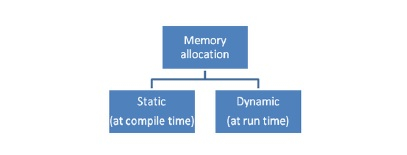# What do you mean by static memory allocation in C programming?

Memory can be allocated in the following two ways −## Static Memory Allocation

Static variable defines in one block of allocated space, of a fixed size. Once it is allocated, it can never be freed.

Memory is allocated for the declared variable in the program.

• The address can be obtained by using ‘&’ operator and can be assigned to a pointer.

• The memory is allocated during compile time.

• It uses stack for maintaining the static allocation of memory.

• In this allocation, once the memory is allocated, the memory size cannot change.

• It is less efficient.

The final size of a variable is decided before running the program, it will be called as static memory allocation. It is also called compile-time memory allocation.

We can't change the size of a variable which is allocated at compile-time.

## Example 1

Static memory allocation is generally used for an array. Let’s take an example program on arrays −

Live Demo

#include<stdio.h>
main (){
int a = {10,20,30,40,50};
int i;
printf (“Elements of the array are”);
for ( i=0; i<5; i++)
printf (“%d, a[i]);
}

## Output

Elements of the array are
1020304050

## Example 2

Let’s consider another example to calculate sum and product of all elements in an array −

Live Demo

#include<stdio.h>
void main(){
//Declaring the array - run time//
int array={10,20,30,40,50};
int i,sum=0,product=1;
//For loop//
for(i=0;i<5;i++){
//Calculating sum and product, printing output//
sum=sum+array[i];
product=product*array[i];
}
//Displaying sum and product//
printf("Sum of elements in the array is : %d",sum);
printf("Product of elements in the array is : %d",product);
}

## Output

Sum of elements in the array is : 150
Product of elements in the array is : 12000000